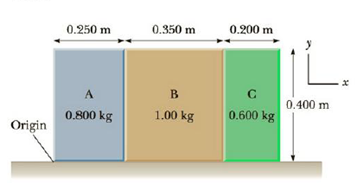Chapter 8, Problem 10P

Chapter
Section
Textbook Problem

Three solid, uniform boxes are aligned as in Figure P8.10. Find the x- and y-coordinates of the center of mass of the three boxes, measured from the bottom left corner of box A.Figure P8.10

To determine
The x -and y -coordinates of the center of mass of the three boxes measured from the bottom left corner of box A .

Explanation

Section1:

To determine: The x co-ordinate of center of mass of the boxes.

Answer: The x co-ordinate of center of mass of the boxes is 0.394m .

Explanation:

Given info: Masses of the boxes A , B , and C are 0.800kg , 1.00kg , and 0.600kg respectively and their center of masses are located at (0.250m)/2 , (0.350m)/2 , and (0.200m)/2 .

The formula for the x co-ordinate of the center of mass of boxes is,

xcm=mAxA+mBxB+mCxCmA+mB+mC

• mA,mB , and mC are masses of the boxes.
• xA,xB , and xC are x coordinates of center of masses of boxes.

Substitute 0.800kg for mA , 1.00kg for mB , 0.600kg for mC , (0.250m)/2 for xA , (0.350m)/2 for xB , and (0.200m)/2 for xC to find xcm

Still sussing out bartleby?

Check out a sample textbook solution.

See a sample solution

The Solution to Your Study Problems

Bartleby provides explanations to thousands of textbook problems written by our experts, many with advanced degrees!

Get Started